• 13.2 Class 10
• Exercise 13.2 Class 10
• Exercise 13.2 Class 10 Maths Solutions
• Class 10 Exercise 13.2 Maths NCERT Solutions

## Excercise: 13.2 Class 10 NCERT Solutions

### Ans: (Exercise 13.1 Class 10) :-

The volume of the solid is given by :

The volume of solid = Volume of cone + Volume of a hemisphere

The volume of cone :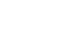or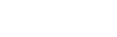or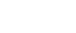And the volume of the hemisphere :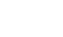or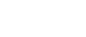or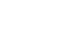Hence the volume of solid is :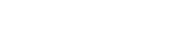### Ans: (Exercise 13.1 Class 10) :-

The volume of air present = Volume of cylinder + 2 (Volume of a cone)

Now, the volume of a cylinder :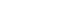or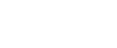or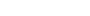And the volume of a cone is :or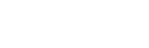orThus the volume of air is :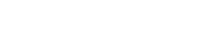or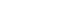#### Q.3 A gulab jamun contains sugar syrup up to about 30% of its volume. Find approximately how much syrup would be found in 45 gulab jamuns, each shaped like a cylinder with two hemispherical ends with length 5 cm and diameter 2.8 cm (see Fig).### Ans: (Exercise 13.1 Class 10) :-

It is clear from the figure that gulab jamun has one cylindrical part and two hemispherical parts.

Thus, the volume of gulab jamun is = Volume of cylindrical part + 2 (Volume of the hemisphere )

Now, the volume of the cylinder isor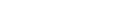or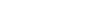And the volume of a hemisphere is :Thus the volume of 1 gulab jamun is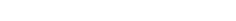Hence the volume of 45 gulab jamun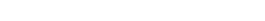Further, it is given that one gulab jamun contains sugar syrup upto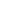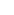.

So, the total volume of sugar present :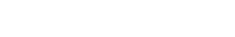#### Q.4 A pen stand made of wood is in the shape of a cuboid with four conical depressions to hold pens. The dimensions of the cuboid are 15 cm by 10 cm by 3.5 cm. The radius of each of the depressions is 0.5 cm and the depth is 1.4 cm. Find the volume of wood in the entire stand (see Fig. 13.16).### Ans: (Exercise 13.1 Class 10) :-

The volume of wood is given by = volume of the cuboid – the volume of four cones.

Firstly, the volume of cuboid :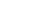or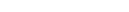or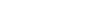And, the volume of cone :Thus the volume of wood is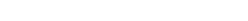#### Q.5 A vessel is in the form of an inverted cone. Its height is 8 cm and the radius of its top, which is open, is 5 cm. It is filled with water up to the brim. When lead shots, each of which is a sphere of radius 0.5 cm are dropped into the vessel, one-fourth of the water flows out. Find the number of lead shots dropped in the vessel.

Ans: (Exercise 13.1 Class 10) :-

According to the question :

Water spilled from the container = Volume of lead balls.

Let us assume the number of lead balls to be n.

Thus the equation becomes :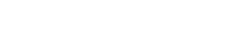or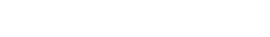or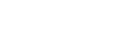or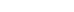Hence the number of lead shots dropped is 100.

### Ans: (Exercise 13.1 Class 10) :-

The pole can be divided into one large cylinder and one small cylinder.

Thus, the volume of pole = volume of large cylinder + volume of a small cylinder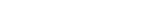or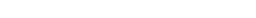or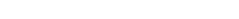or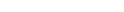or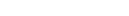Now, according to question mass of the pole is :or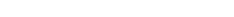### Ans: (Exercise 13.1 Class 10) :-

It is clear from the question that the required volume is :

The volume of water (left) =Volume of a cylinder – Volume of solid

Now the volume of the cylinder isor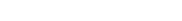And the volume of solid is :Thus the volume of water left :### Ans: (Exercise 13.1 Class 10) :-

The volume of the vessel is given by :

The volume of vessel = Volume of sphere + Volume of the cylindrical part

Now, the volume of the sphere is :And the volume of the cylinder is:-Thus the volume of the vessel is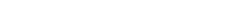#### More About NCERT Solutions for Class 10th Maths Exercise 13.2:-

NCERT Solutions for Class 10th Maths exercise 13.2- The most important part of NCERT Solutions for Class 10th Maths chapter 13 Exercise 13.2 is that it helps you to determine how to break the figure in those parts or shapes with which we are familiar and we know their formulas. For example – In the question given in number 2 of exercise 13.2, we have broken the shape using two cones and one cylinder because we are familiar with these shapes. We should also know different formulas like formulas for finding the volume of cones, cylinders etc.

Some important formulas are:

Volume of cube =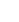Volume of cylinder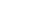Benefits of NCERT Solutions for Class 10 Maths Exercise 13.2

• Exercise 13.2 Class 10 Maths, is based on Volume of a Combination of Solids.

• Class 10 maths chapter 13 exercise 13.2 helps us in understanding complex shapes and finding its volume.

• Class 10th maths chapter 13 exercise 13.2 helps us to simplify complex shapes by breaking it into smaller individual objects that are simple to our understanding.

## Frequently Asked Question (FAQs) – NCERT Solutions for Exercise 13.2 Class 10 Maths Chapter 13 – Surface Area and Volumes

### Q:-What is the core concept of NCERT solutions for class 10 maths exercise 13.2?

Ans: (Exercise 13.1 Class 10) :-

The core concept behind this exercise is to find the volume combination of solids. We have to remember the formula for finding the volume of cuboid, sphere, cylinder etc

Q:- Write the formula for finding the volume of cone, cylinder and sphere?

Ans: (Exercise 13.1 Class 10) :-

Cone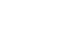Cylinder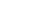Sphere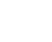### Q:-If a cone and a hemisphere of same radius are placed on one another such that the hemisphere lies in the bottom of the arrangement, find the total volume of the arrangement?

Ans: (Exercise 13.1 Class 10) :-

Total volume = volume of cone + volume of hemisphere

Total volume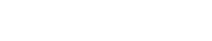(Where is the r radius of the cone and h is the height of the cone)

### Q:-If a cylinder and a hemisphere of same radius are placed on one another such that the cylinder lies in the bottom of the arrangement, find the total volume of the arrangement?

Ans: (Exercise 13.1 Class 10) :-

Total volume = volume of cylinder + volume of hemisphere

Total volume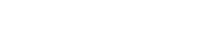(Where is the r radius of the cylinder and h is the height of the cylinder)

Q:- How many cubes of side ‘x’ is required to make a new cube of side ‘2x’?

Ans: (Exercise 13.1 Class 10) :-

Our initial volume is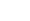Out final volume is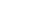No of cubes required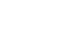Q:- A cube of ‘x’ is placed below a sphere such that the diameter of the sphere is equal to the diagonal of the face of the cube. Find the total volume of the arrangement?

Ans: (Exercise 13.1 Class 10) :-

Since the diameter is equal to the diagonal of the face of the cube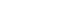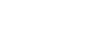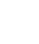Where is the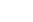diagonal of the cube and r is the radius of the sphere

Now we replace the radius of the sphere by

Total volume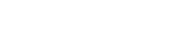Total volume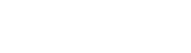### Q:-A hemisphere is removed from the bottom of a cylinder of radius ‘r’ and height ‘3r’ find the volume of the remaining portion?

Ans: (Exercise 13.1 Class 10) :-

Total volume = volume of the cylinder – the volume of the hemisphere

Volume of cylinderVolume of hemisphereTotal volume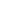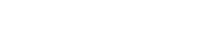Now h=3r

Total volume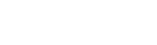EXERCISE 13.2
1 . A solid is in the shape of a cone standing on a hemisphere with both their radii being
equal to 1 cm and the height of the cone is equal to its radius. Find the volume of the solid
in terms of π.

2. Rachel, an engineering student, was asked to make a model shaped like a cylinder with
two cones attached at its two ends by using a thin aluminium sheet. The diameter of the
model is 3 cm and its length is 12 cm. If each cone has a height of 2 cm, find the volume
of air contained in the model that Rachel made. (Assume the outer and inner dimensions
of the model to be nearly the same.)

3. A gulab jamun, contains sugar syrup up to about
30% of its volume. Find approximately how much
syrup would be found in 45 gulab jamuns, each
shaped like a cylinder with two hemispherical ends

4. A pen stand made of wood is in the shape of a
cuboid with four conical depressions to hold pens.
The dimensions of the cuboid are 15 cm by 10 cm by
3.5 cm. The radius of each of the depressions is 0.5
cm and the depth is 1.4 cm. Find the volume of
wood in the entire stand (see Fig. 13.16).

5. A vessel is in the form of an inverted cone. Its
height is 8 cm and the radius of its top, which is
open, is 5 cm. It is filled with water up to the brim.
0.5 cm are dropped into the vessel, one-fourth of
the water flows out. Find the number of lead shots
dropped in the vessel.

6. A solid iron pole consists of a cylinder of height 220 cm and base diameter 24 cm, which
is surmounted by another cylinder of height 60 cm and radius 8 cm. Find the mass of the
pole, given that 1 cm3 of iron has approximately 8g mass. (Use π = 3.14)

7. A solid consisting of a right circular cone of height 120 cm and radius 60 cm standing on
a hemisphere of radius 60 cm is placed upright in a right circular cylinder full of water
such that it touches the bottom. Find the volume of water left in the cylinder, if the radius
of the cylinder is 60 cm and its height is 180 cm.

8. A spherical glass vessel has a cylindrical neck 8 cm long, 2 cm in diameter; the diameter
of the spherical part is 8.5 cm. By measuring the amount of water it holds, a child finds its
volume to be 345 cm3.